### Home > A2C > Chapter Ch12 > Lesson 12.1.3 > Problem12-53

12-53.
1. Solve each of the following equations without a calculator.
Homework Help ✎

1. logx 17 = 1

2.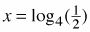3.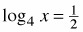4. log3 81 = x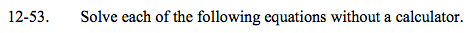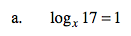x = 17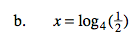$4^x = \frac{1}{2}$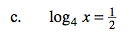$\small x=4^{\frac{1}{2}}=2$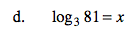See part (b).